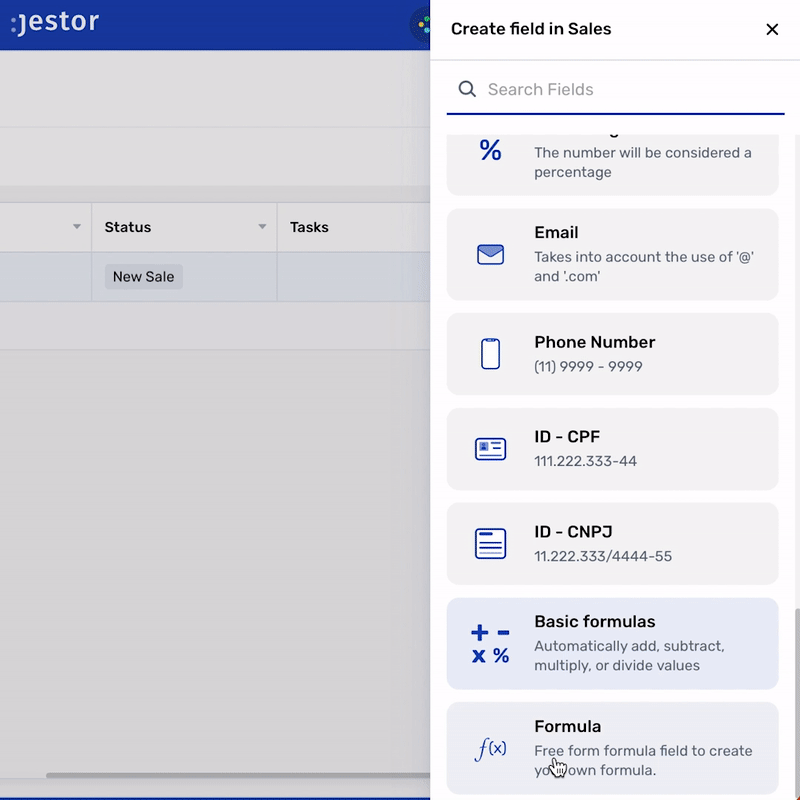# Formula

Free form formula field to create your own formula.

# What is the Formula field?

The Formula Field supports both basic operators and complex functions. You can use fixed numbers, fields of the record itself and even fields from connected records to build your formula!# Functions

Functions are predetermined complex operations that will return you a value. The ones currently supported are explained below:

IF: returns a value if a condition is true and a different value if it's false.

CONCAT: allows you to concatenate text, like combining pine and apple to return pineapple.

ROUND: rounds a number to a given number of decimal places.

DATE_SUB(date, INTERVAL value interval)

MICROSECOND(datetime)

SECOND(datetime)

MINUTE(time)

HOUR(time)

DAY(date)

MONTH(date)

YEAR(date)

DATEDIFF(date1, date2)

DATE(expression)

DATE_FORMAT(date, format):

TIME_FORMAT(time, format):

NOW()

### 🚧Parentheses

You can use parenthesis to define the order of operations, for example: ( {{pre_total_2}}*{{amount}} ) - {{discount_2}}

# Basic operators

Addition, Subtraction, Division and Multiplication. classic operators you can use along with functions.

Subtraction: -
Division: /
Multiplication: *

# How to create a Formula field

To create a Formula field:

• On table mode, click on the last table header with a "+" and that will open the create field page
• Search for the "Formula" option and click on it
• Choose a name for your field and write the desired formula

### 📘

You can delete or edit by clicking on the table header name and choosing one of the options